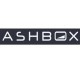﻿ RGB to HEX in Excel | Adam Dimech's Coding Blog

Dear Internet Explorer user: Your browser is no longer supported

Please switch to a modern browser such as Microsoft Edge, Mozilla Firefox or Google Chrome to view this website's content.

# RGB to HEX in Excel

Use Microsoft Excel to convert Red-Green-Blue (RGB) values into hexadecimal colour codes and display the relevant colour graphically beside each output.

The aim of this tutorial is to demonstrate how Microsoft Excel can be used to illustrate colours graphically, by converting codes from the RGB Colour Model into hexadecimal colour codes and then displaying the matching colour beside each.

I recently needed this functionality to allow me to describe colour for written reports, but there are many applications for such a utility.

## Step 1: Set up the Excel sheet

Set up a new Excel sheet with columns named as follows:

• Column A = “R”
• Column B = “G”
• Column C = “B”
• Column D = “Hex”
• Column E = “Colour”

When complete, this worksheet will enable you to enter RBG values into the first three columns and then the HEX code will be output in Column D with a colour sample in Column E.

## Step 2: Format as table and enter data

On the worksheet, select a range of cells that you want to quickly format as a table. On the Home tab, in the Styles group, click Format as Table.

Enter RBG values into the first three columns and save the workbook in XLSM (Excel Macro-Enabled Workbook) format.

## Step 3: Derive HEX values

In the second row of Column 4 (“HEX”), insert the following formula:

=IF(ISODD(LEN(DEC2HEX([@R]))),"0","")&DEC2HEX([@R])&IF(ISODD(LEN(DEC2HEX([@G]))),"0","")&DEC2HEX([@G])&IF(ISODD(LEN(DEC2HEX([@B]))),"0","")&DEC2HEX([@B])

HEX values should now be displayed to match each RGB combination entered. The formula uses the DEC2HEX function to convert RGB values into HEX codes, but needs to be further modified in order to allow for leading zeroes.

## Step 4: Insert the VBA scripts

Press Alt + F11 or otherwise go to the Developer ribbon and choose the “Visual Basic” icon if the Developer Tab is enabled. In the Visual Basic for Applications toolbar, go to Insert > Module

Paste the following code (based on the work of “pnuts“):

Sub ColourMyCells()

Dim LastRow As Integer
On Error Resume Next
LastRow = ActiveSheet.UsedRange.Rows.Count
On Error GoTo 0
If LastRow = 0 Then
Exit Sub
Else
Dim i As Integer
For i = 2 To LastRow
Cells(i, 5).Interior.Color = RGB(Cells(i, 1), Cells(i, 2), Cells(i, 3))
Next i
End If
End Sub

Next, double-click on “Sheet1” under “Microsoft Excel Objects” and paste in the following code:

Private Sub Worksheet_Change(ByVal Target As Range)
ColourMyCells
End Sub


This second code is for a worksheet change event that tells Visual Basic to execute the ColourMyCells script whenever any change is made to a cell in Sheet1.

The Excel sheet should now display colours beside the HEX codes.

14 responses to “RGB to HEX in Excel”RGB HEX Converter, is a fast app to convert units of color: RGB to HEX, very useful for graphic designers and web designersHi,

This is really useful to me right now, but I can´t get it to work…
First, Excel doesn’t let me name column A to R, says it’s not a valid name or in use by an object (even on a brand new file), and the the same with the formula.. says it´s not valid.

I´m on Excel 2010.

Any ideas why?Worked perfectly, once I got the hex strings translated successfully – your syntax returned a name error on my machine, for the R, G and B cell referents. So I wrote my own @Left, @Mid and @Right text extracts in a second sheet and then just referenced the result as Sheet2!G2 etc in D2 et al.
The Range Names appear correct, no spaces etc?
Many thanks.This is the very basic concept you have capture here. Whenever I in need of color code with the perfect scale I prefer to use online color code converter tools. I produce your code and I am amazed that it work successfully.This took my time but I come to know about the process of color code conversion. Can we proceed it in decimal or octal code?Hello, great explanation! I have understood everything! Buy just a question, How you have converted Decimal to binary in this example? Did you use big numbers javascript package for the same? In the above example they have used Bignumbers package.The DeEC2HEX function allows for a second argument, which you should set to ‘2’.

The new formula could then simply be:

=DEC2HEX( [R] ,2) & DEC2HEX( [G] ,2) & DEC2HEX( [B] ,2)Dear Spanish People.. this is the formula in spanish… =SI(ES.IMPAR(LARGO(DEC.A.HEX([@R])));”0″;””)&DEC.A.HEX([@R])&SI(ES.IMPAR(LARGO(DEC.A.HEX([@G])));”0″;””)&DEC.A.HEX([@G])&SI(ES.IMPAR(LARGO(DEC.A.HEX([@B])));”0″;””)&DEC.A.HEX([@B])I too am having the naming problem of WalterT. I don;t kniw my way around as well, can someone tell me how to change the code to reference the columns correctly, or the column names? I ahve tried changing them to text and that doesn’t work. Thanks, SharonThis is the formula in PT-BR:
=SE(ÉIMPAR(NÚM.CARACT(DECAHEX(TabelaRGB[@R])));”0″;””)&DECAHEX(TabelaRGB[@R])&SE(ÉIMPAR(NÚM.CARACT(DECAHEX(TabelaRGB[@G])));”0″;””)&DECAHEX(TabelaRGB[@G])&SE(ÉIMPAR(NÚM.CARACT(DECAHEX(TabelaRGB[@B])));”0″;””)&DECAHEX(TabelaRGB[@B])I followed all the instructions but only ColurMyCells Warks. The formula convert to Hex doesn’t works and return me a strange error message not recognising the string as a formula.
The message is:
“There is a problem with this formula. Not trying to type a formula? When the first character is an equal (“=”) or minus (“-“) sign, Excel thinks it’s a formula: you type =1+1, cell shows: 2.”
I copied and pasted the strings as in this page : =IF(ISODD(LEN(DEC2HEX([@R]))),”0″,””)&DEC2HEX([@R])&IF(ISODD(LEN(DEC2HEX([@G]))),”0″,””)&DEC2HEX([@G])&IF(ISODD(LEN(DEC2HEX([@B]))),”0″,””)&DEC2HEX([@B])
Everything else is working and I’m using the latest version on Excel for Mac. Any idea?Just cannot get it to work…. Need to convert about 2000 rgb values to hex. Anyone has a ready to go file for download?

<a href="" title=""> <b> <blockquote cite=""> <code> <em> <i> <q cite=""> <strike> <strong>